## Electric Power System (Model of Dobson and Chiang)

exd15
Variables and parameters:

V: magnitude of the load voltage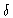: phase angle of V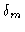: generator voltage phase angle: rotor speed

Q1: reactive power demand (bifurcation parameter: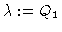)

Model equations: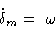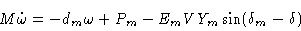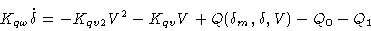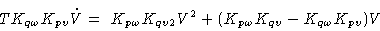with variables and constants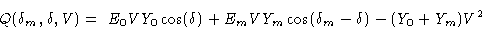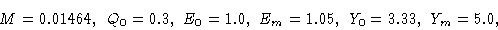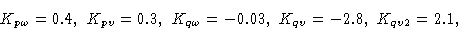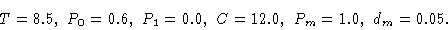Figure 1
Bifurcation diagram V versus load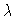(detail: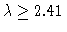, all stationary states and some periodic states; for the latter ones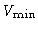is depicted).

Figure 2
Bifurcation diagram V andversus load. detail: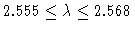, all stationary states and some periodic states (S: stationary, P: periodic, s: stable, u: unstable, HB: Hopf bifurcation, PD: periodic doubling)

Figure 3
Phase diagram (projection). Horizontal axis:; vertical axis: V. (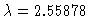)

Figure 4
Phase diagram (projection). Horizontal axis:; vertical axis: V. (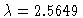)

Figure 5
Phase diagram (projection). Horizontal axis:; vertical axis: V. (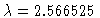)

Figure 6
Phase diagram, periodic orbit, unstable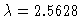, T=4.6376, projection: horizontal axis, vertical axis V.

Figure 7
Phase diagram, two periodic orbits for, one stable (T=3.802) and one unstable (T=1.913) projection: horizontal axis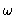, vertical axis V.

Figure 8
Phase diagram, unstable periodic orbit,, T=7.62, projection: horizontal axis, vertical axis V.

Figure 9
Phase diagram,,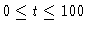. projection: horizontal axis, vertical axis V.

Figure 10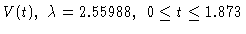. Periodic orbit: simple'' period.

Figure 11. Periodic orbit: double'' period.

Figure 12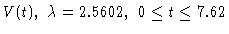. Periodic orbit: fourfold'' period.

Figure 13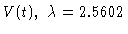Figure 14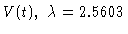Figure 15
V(t), withvarying linearly from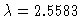to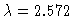. Collapse of voltage.

Figure 16
Phase diagram corresponding to previous figure. Projection to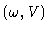-plane,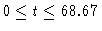, withvarying linearly fromto.

This Example
Figure 1
Figure 2
Figure 3
Figure 4
Figure 5
Figure 6
Figure 7
Figure 8
Figure 9
Figure 10
Figure 11
Figure 12
Figure 13
Figure 14
Figure 15
Figure 16